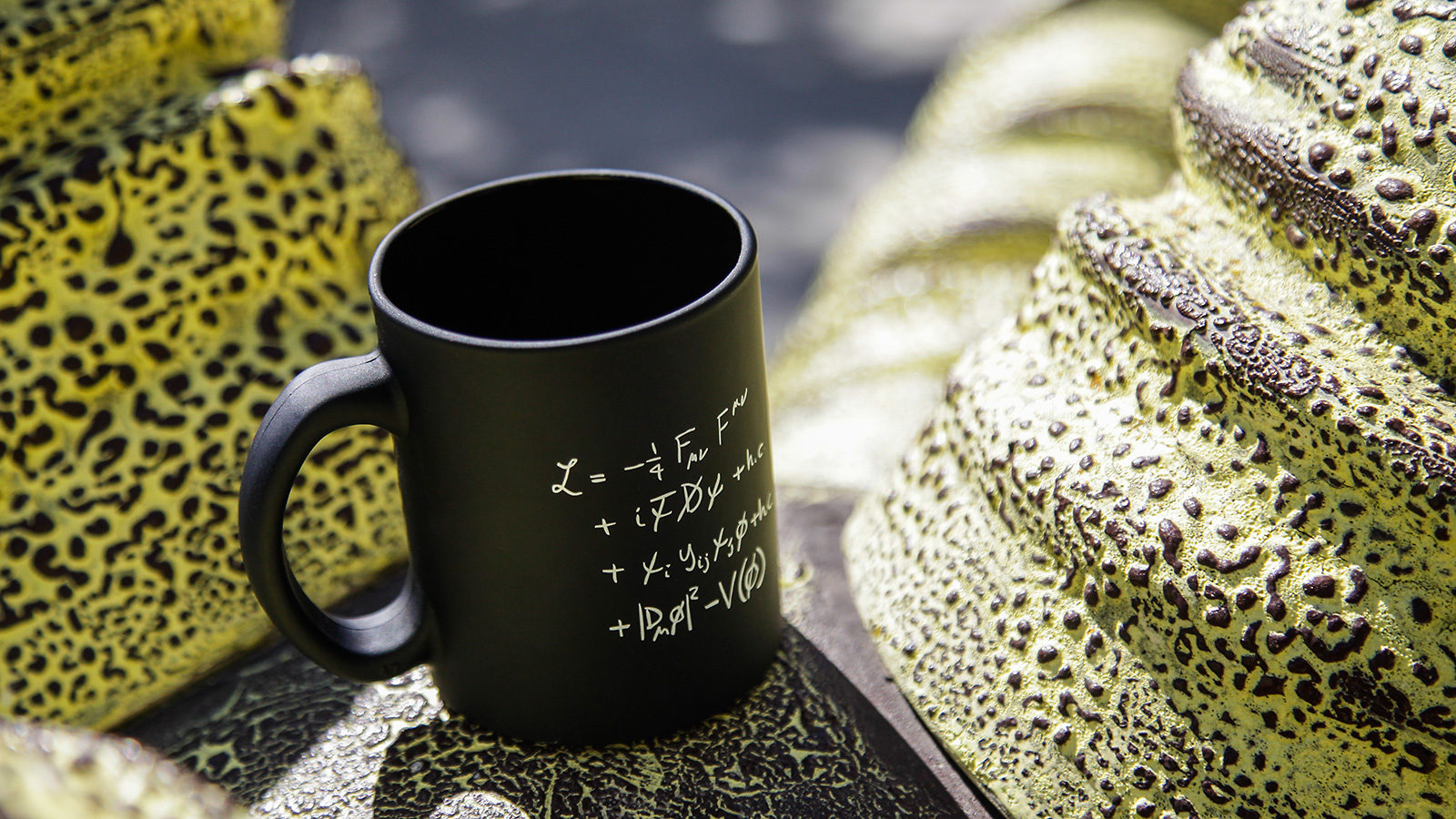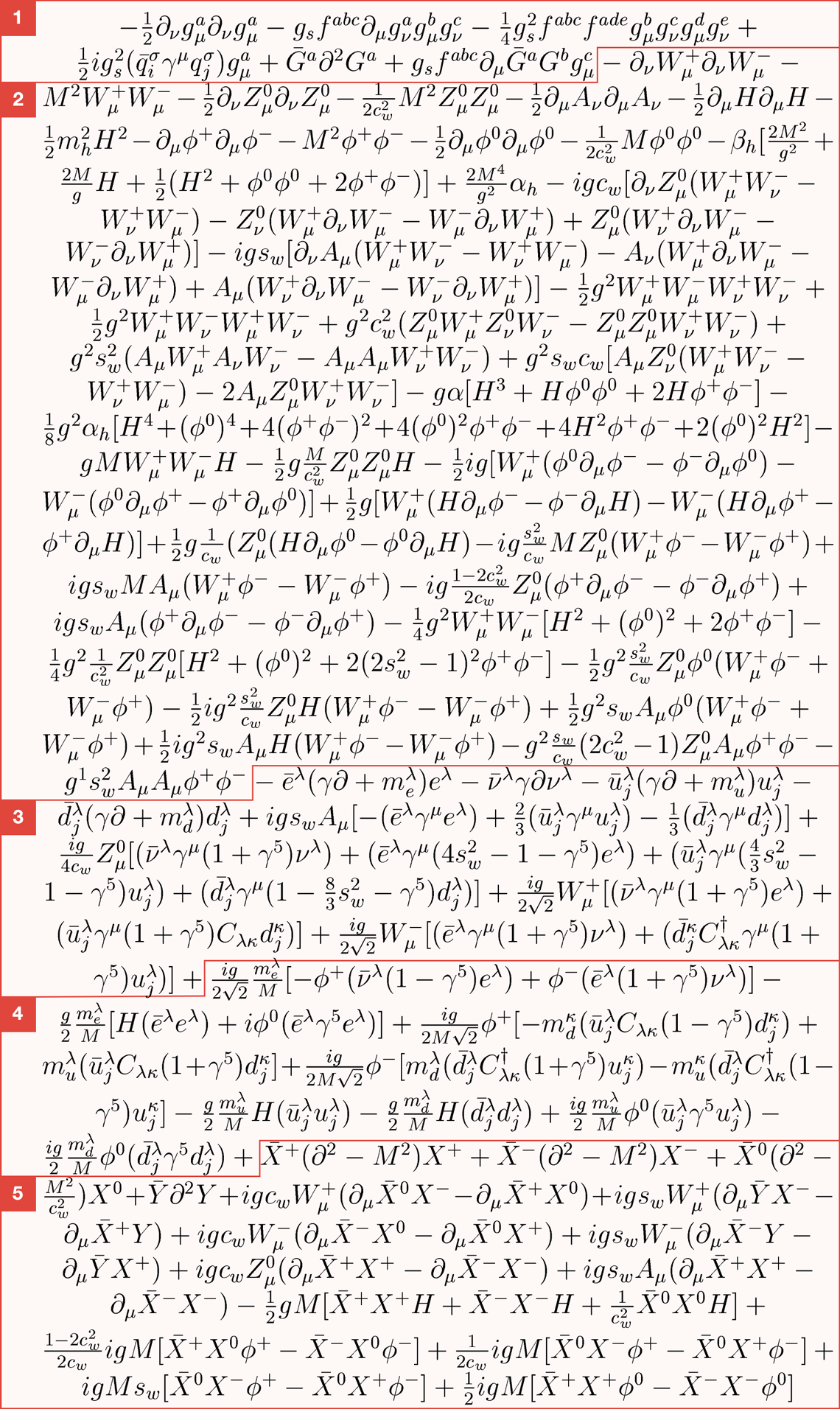A joint Fermilab/SLAC publicationYvonne Tang, SLAC National Accelerator Laboratory

# The deconstructed Standard Model equation

07/28/16

The Standard Model is far more than elementary particles arranged in a table.

The Standard Model of particle physics is often visualized as a table, similar to the periodic table of elements, and used to describe particle properties, such as mass, charge and spin. The table is also organized to represent how these teeny, tiny bits of matter interact with the fundamental forces of nature.

But it didn’t begin as a table. The grand theory of almost everything actually represents a collection of several mathematical models that proved to be timeless interpretations of the laws of physics.

Here is a brief tour of the topics covered in this gargantuan equation.

### The whole thing

This version of the Standard Model is written in the Lagrangian form. The Lagrangian is a fancy way of writing an equation to determine the state of a changing system and explain the maximum possible energy the system can maintain.

Technically, the Standard Model can be written in several different formulations, but, despite appearances, the Lagrangian is one of the easiest and most compact ways of presenting the theory.Lagrangian standard model

Courtesy of T.D. Gutierrez

### Section 1

These three lines in the Standard Model are ultraspecific to the gluon, the boson that carries the strong force. Gluons come in eight types, interact among themselves and have what’s called a color charge.

### Section 2

Almost half of this equation is dedicated to explaining interactions between bosons, particularly W and Z bosons.

Bosons are force-carrying particles, and there are four species of bosons that interact with other particles using three fundamental forces. Photons carry electromagnetism, gluons carry the strong force and W and Z bosons carry the weak force. The most recently discovered boson, the Higgs boson, is a bit different; its interactions appear in the next part of the equation.

### Section 3

This part of the equation describes how elementary matter particles interact with the weak force. According to this formulation, matter particles come in three generations, each with different masses. The weak force helps massive matter particles decay into less massive matter particles.

This section also includes basic interactions with the Higgs field, from which some elementary particles receive their mass.

Intriguingly, this part of the equation makes an assumption that contradicts discoveries made by physicists in recent years. It incorrectly assumes that particles called neutrinos have no mass.

### Section 4

In quantum mechanics, there is no single path or trajectory a particle can take, which means that sometimes redundancies appear in this type of mathematical formulation. To clean up these redundancies, theorists use virtual particles they call ghosts.

This part of the equation describes how matter particles interact with Higgs ghosts, virtual artifacts from the Higgs field.

### Section 5

This last part of the equation includes more ghosts. These ones are called Faddeev-Popov ghosts, and they cancel out redundancies that occur in interactions through the weak force.

Note: Thomas Gutierrez, an assistant professor of Physics at California Polytechnic State University, transcribed the Standard Model Lagrangian for the web. He derived it from Diagrammatica, a theoretical physics reference written by Nobel Laureate Martinus Veltman. In Gutierrez’s dissemination of the transcript, he noted a sign error he made somewhere in the equation. Good luck finding it!